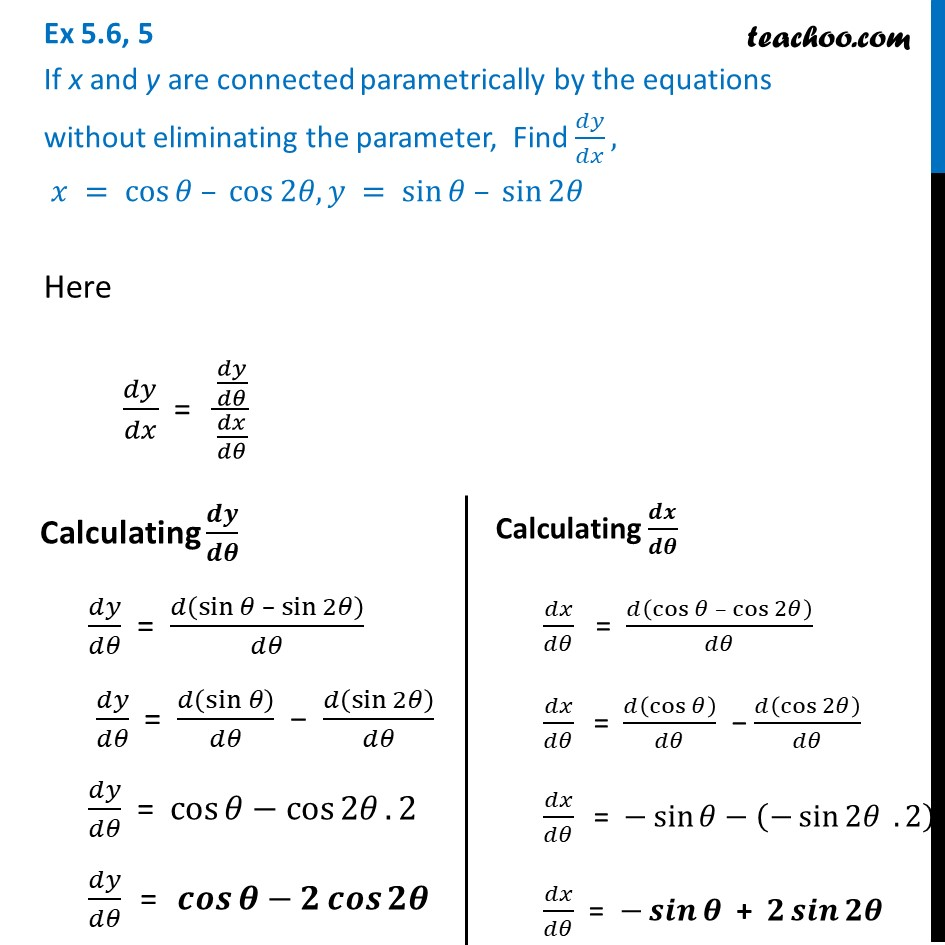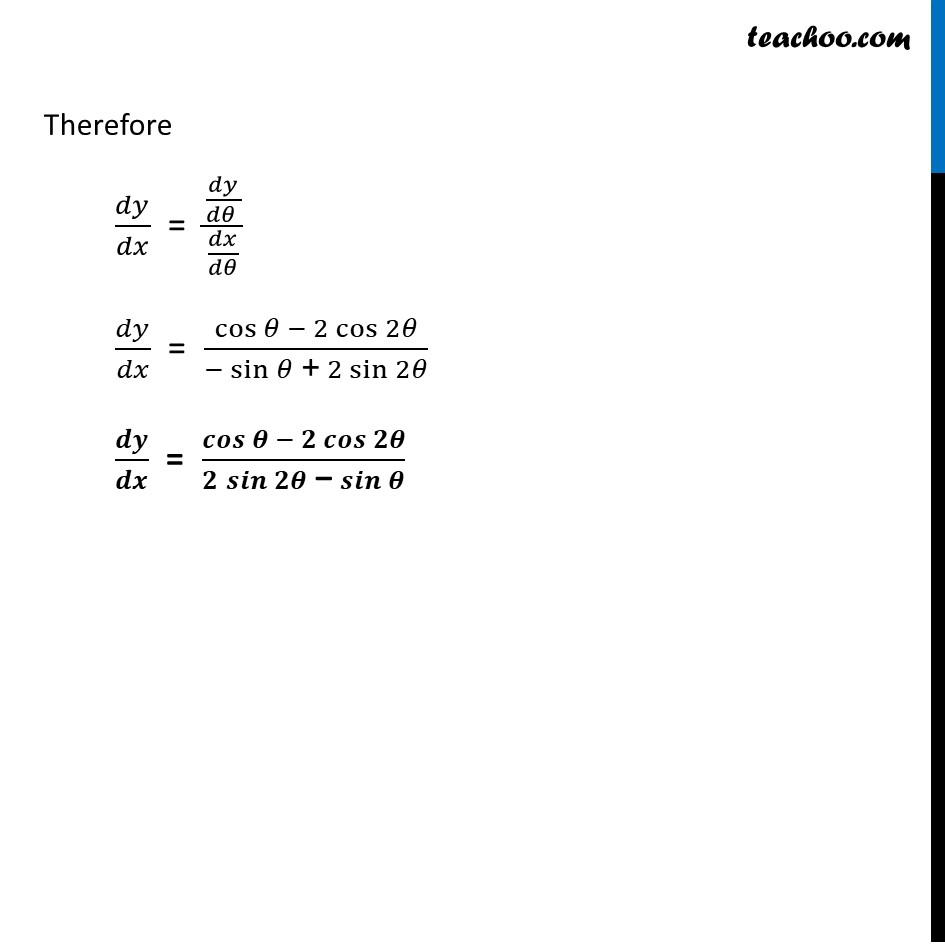Ex 5.6

Chapter 5 Class 12 Continuity and Differentiability
Serial order wiseLearn in your speed, with individual attention - Teachoo Maths 1-on-1 Class

### Transcript

Ex 5.6, 5 If x and y are connected parametrically by the equations without eliminating the parameter, Find 𝑑𝑦/𝑑𝑥, 𝑥 = cos⁡𝜃 – cos⁡2𝜃, 𝑦 = sin⁡𝜃 – sin⁡2𝜃 Here 𝑑𝑦/𝑑𝑥 = (𝑑𝑦/𝑑𝜃)/(𝑑𝑥/𝑑𝜃) Calculating 𝒅𝒚/𝒅𝜽 𝑑𝑦/𝑑𝜃 = 𝑑(sin⁡𝜃 – sin⁡2𝜃)/𝑑𝜃 𝑑𝑦/𝑑𝜃 = 𝑑(sin 𝜃)/𝑑𝜃 − 𝑑(sin⁡2𝜃)/𝑑𝜃 𝑑𝑦/𝑑𝜃 = cos⁡𝜃−cos⁡2𝜃. 2 𝑑𝑦/𝑑𝜃 = 𝒄𝒐𝒔⁡𝜽−𝟐 𝒄𝒐𝒔⁡𝟐𝜽 Calculating 𝒅𝒙/𝒅𝜽 𝑑𝑥/𝑑𝜃 = 𝑑(cos⁡𝜃 – cos⁡2𝜃)/𝑑𝜃 𝑑𝑥/𝑑𝜃 " " = 𝑑(cos⁡𝜃)/𝑑𝜃 − 𝑑(cos⁡2𝜃)/𝑑𝜃 𝑑𝑥/𝑑𝜃 " " = −sin⁡𝜃−(−sin⁡2𝜃 . 2) 𝑑𝑥/𝑑𝜃 = −𝒔𝒊𝒏⁡𝜽 + 𝟐 𝒔𝒊𝒏⁡𝟐𝜽 Therefore 𝑑𝑦/𝑑𝑥 = (𝑑𝑦/(𝑑𝜃 ))/(𝑑𝑥/𝑑𝜃) 𝑑𝑦/𝑑𝑥 = (cos⁡𝜃 − 2 cos⁡2𝜃)/(−sin⁡𝜃 " +" 2 sin⁡2𝜃 ) 𝒅𝒚/𝒅𝒙 = (𝒄𝒐𝒔⁡𝜽 − 𝟐 𝒄𝒐𝒔⁡𝟐𝜽)/(𝟐 𝒔𝒊𝒏⁡𝟐𝜽 " −" 𝒔𝒊𝒏⁡𝜽 )